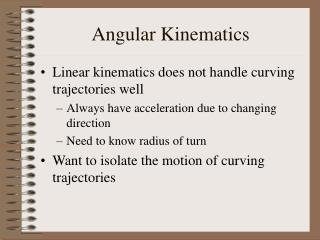DownloadDownload PresentationAngular Kinematics

Angular Kinematics

Télécharger la présentationAngular Kinematics

- - - - - - - - - - - - - - - - - - - - - - - - - - - E N D - - - - - - - - - - - - - - - - - - - - - - - - - - -
Presentation Transcript

1. Angular Kinematics • Linear kinematics does not handle curving trajectories well • Always have acceleration due to changing direction • Need to know radius of turn • Want to isolate the motion of curving trajectories

2. A straight line has an always changing “radius” from a single point • Can think of “rolling up” a line so the radius is constant

3. Definition of angular quantities • Angle corresponds to displacement • q = x / r • x is distance along curve, r is radius of curve • Angular velocity corresponds to velocity • w = Dq/Dt = v / r • Angular acceleration corresponds to tangential acceleration • a = Dw/Dt = at / r • at generates a speed change, not a directional change

4. Note that these are simply the linear quantities divided by the radius! • Can we use this to find kinematic relationships? • This is the displacement equation for angular motion!

5. Can derive other kinematic equations in a same way • Notice that linear kinematic equation and angular kinematic equation are almost the same • Replace linear quantities with angular quantities to transform between them

6. Direction of angular motion • Angular motion, like all motion, has a direction • Only stationary point is the center of the circle • Center defines axis of rotation • Use right hand rule to define direction • Curl fingers of right hand in direction of spin • Thumb points in direction of motion